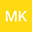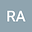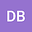On new analytical and semi-analytical wave solutions of the quadratic-cubic fractional nonlinear Schrodinger equation
•••• mostafa Khater,
• Raghda Attia,
• Dumitru Baleanu
mostafa Khater
Jiangsu University
Author ProfileRaghda Attia
Jiangsu University
Author ProfileDumitru Baleanu
Cankaya Universitesi
Author Profile## Abstract

This research paper discusses the analytical and semi-analytical solutions of the quadratic–cubic fractional nonlinear Schrodinger (NLS) equation. By applying a new fractional operator we transform the fractional formula of the model to integer-order, which allows applying the analytical and numerical methods on it. The analytical solutions are obtained by the implementation of two distinct systematic schemes and the reported solutions are used in applying the Adomian decomposition method to get the semi-analytical wave solutions of this model. These solutions are used to characterize the changes over time of a physical system in which case of quantum influence, such as wave-particle duality. The comparison between the analytical and semi-analytical solutions are given to explain the accuracy of the obtained solutions.